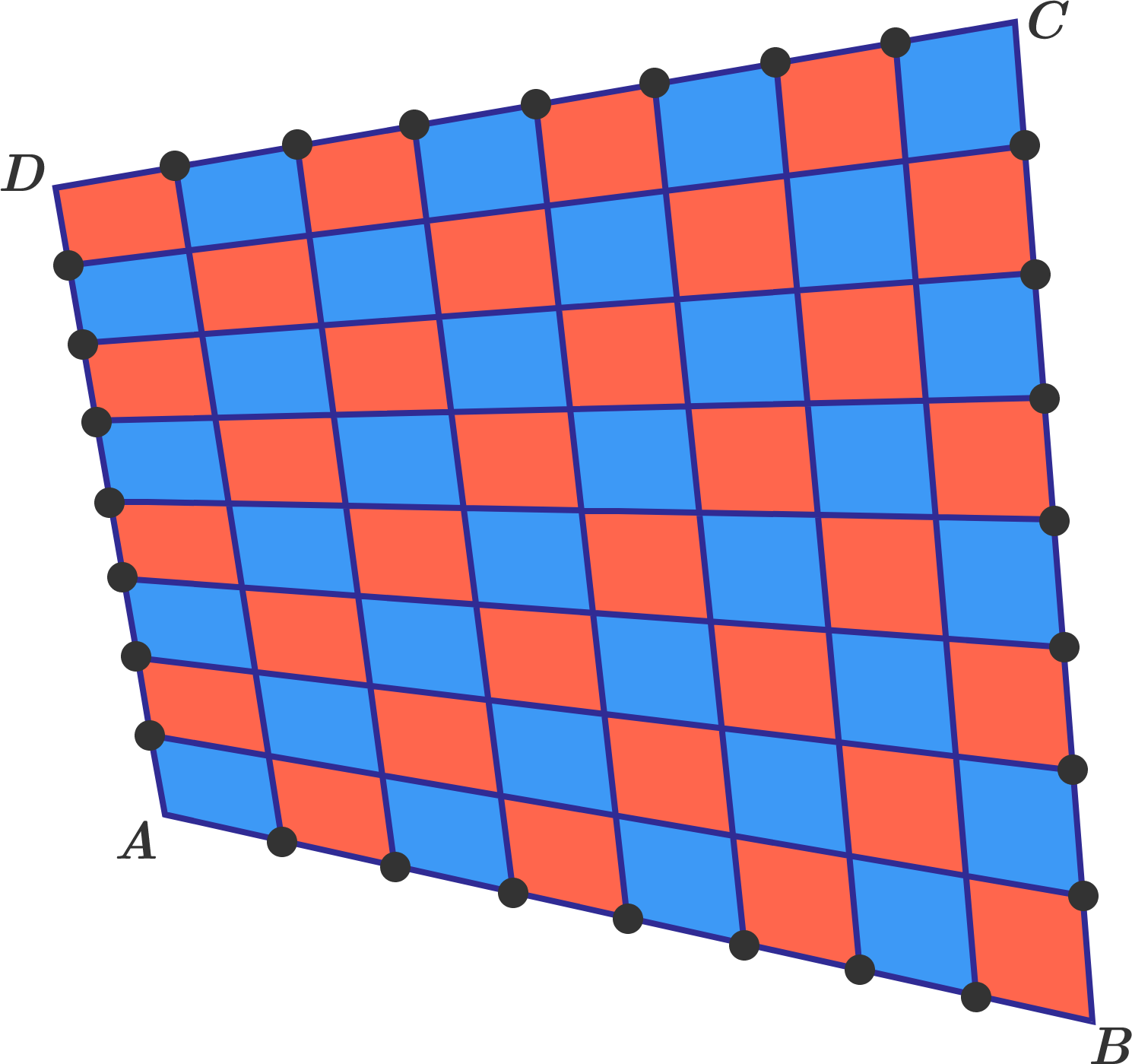# Relative Area Of A Quadrilateral

Geometry Level 1

$ABCD$ is a quadrilateral with known side lengths $AB=2016, BC=2017, CD=2018$. The black points divide each side of the quadrilateral into eight equal parts.Which area is bigger, the sum of the red quadrilaterals or the sum of the blue ones?

×